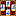###Scoring variations

#### Settling tables – Overview

Four Winds 2 supports three scoring systems that use a settling table to determine the final score for the hand.

Faan-Laak scoring system uses a simple look-up table where a certain amount faans (merits for the hand) corresponds a specified amount of final points. Laaks are multiples of limit points, the doubling of which is regulated by the settling table.

Mangan scoring system determines the final score by using the combination of basic tile points (fu) and doubling factors (han) as the criteria for final score.

Regulated doubles system uses a simple look-up table where a certain amount doubles corresponds a specified amount of multipliers. The multipliers thus achieved are used to multiply the total point scores earned by the hand.

To enable the use of settling table:

1. Choose Preferences on the File menu.
2. From the Rule Presets list, choose a customized rule preset.
3. Choose Payments under the Scoring section of the category tree.
4. From the Use settling table for final scores list, choose one of the following options:
None. The final score is determined directly by calculating the total score of the hand.
Faan-Laak. Specifies that the final score is determined according to the Faan-Laak scoring system.
Mangan. Specifies that the final score is determined according to the Japanese Mangan scoring system.
Regulated doubles. Specifies that the final score is determined by multiplying the basic points score with regulated doubles.
5. If you want to customize the settling table, click the Customize button.

Variations related to settling tables:
Faan-Laak scoring
Auxiliary points
Mangan scoring
Regulated doubles